# Prediction of Brain Stroke Severity Using Machine Learning

Prediction of Brain Stroke Severity Using Machine Learning
Department of Computer Science and Multimedia, Lincoln University College, Kuala Lumpur 47301, Malaysia

Department of Computer Science and Engineering, K L Deemed to be University, KLEF, Guntur 522502, India

Corresponding Author Email:
vamsi.bandi@lincoln.edu.my
Page:
753-761
|
DOI:
https://doi.org/10.18280/ria.340609
2 November 2020
|
Revised:
1 December 2020
|
Accepted:
10 December 2020
|
Available online:
31 December 2020
| Citation

OPEN ACCESS

Abstract:

In recent years strokes are one of the leading causes of death by affecting the central nervous system. Among different types of strokes, ischemic and hemorrhagic majorly damages the central nervous system. According to the World Health Organization (WHO), globally 3% of the population are affected by subarachnoid hemorrhage, 10% with intracerebral hemorrhage, and the majority of 87% with ischemic stroke. In this research work, Machine Learning techniques are applied in identifying, classifying, and predicting the stroke from medical information. The existing research is limited in predicting risk factors pertained to various types of strokes. To address this limitation a Stroke Prediction (SPN) algorithm is proposed by using the improvised random forest in analyzing the levels of risks obtained within the strokes. This research of the Stroke Predictor (SPR) model using machine learning techniques improved the prediction accuracy to 96.97% when compared with the existing models.

Keywords:

intracerebral hemorrhagic stroke, ischemic stroke, improvised random forest, machine learning, stroke prediction, subarachnoid hemorrhagic stroke

1. Introduction

According to “United States centers for Disease Control and Prevention” around 7,95,000 people have been affected with strokes in the year 2018 . On the other hand, when compared with Canadian statistics the overall death rate was around 15,409 people affected with stroke in the year 2000 . Likewise, the statistical report in India given by “India Collaborative Acute Stroke Study” shows 2,162 people are affected with strokes in the year 2004 . As per records of “Stroke Association United Kingdom”, every 5 children out of 100,000 are affected by stroke in the year 2012 .

1.1 Brain stoke

In an adult human being even when the body is set to rest 20% of the oxygen and glucose also about 2% of the entire body weight is constituted by the brain. The blood flow in the brain is said to extend when recreation of the neurons takes palace in certain parts of the brain. It is carried out through the internal carotid and vertebral arteries. The blood is then altered from head to heart through the internal jugular veins .

The loss of the blood might be observed in two scenarios in which the flow of blood among the blood tissues decreases results in the ischemic stroke whereas if internal bleeding occurs among the brain tissues known as hemorrhagic stroke  and the types of strokes are demonstrated in Figure 1.

The flow of the blood from arteries among the brain tissues leads to blockage or becomes slender [7, 8]. This blocking might also happen when the tiny parts of plaque that are caused through atherosclerosis damage thereby creating a clot in the blood vessel [9, 10]. Hemorrhagic stroke referred to as a severe stroke in which the blocked artery might damage resulting in the bleeding or explosion of the artery [11, 12]. When the blood is divulged and leaked it spreads out causing pressure on the brain .

## 1.jpg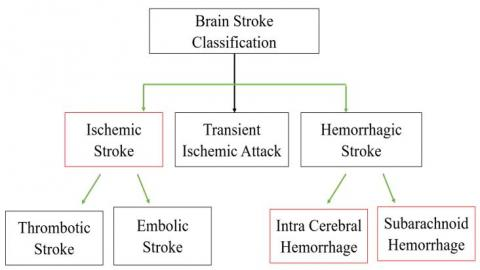Figure 1. Stroke classification

1.2 Machine learning

Machine learning is a study of Artificial Intelligence (AI) and it is a part of computer science, it varies with different perspectives from traditional computations. Machine learning has a feature that permits the system to develop and control the data sets in producing the output values within the limited range . In this technology, the algorithms are defined in the precise way of representing the data sets utilized by the computer in the evaluation of the problems. These algorithms can be implemented through supervised learning and unsupervised learning as shown in Figure 2 . Supervised learning involves the data that is inputted by the humans by retrieving the concerning output. Whereas the backend part of running the algorithm for implementing the accurate results can be done through unsupervised learning without the labeled data by allowing it to find the architecture based upon the input given by the user.

## 2.png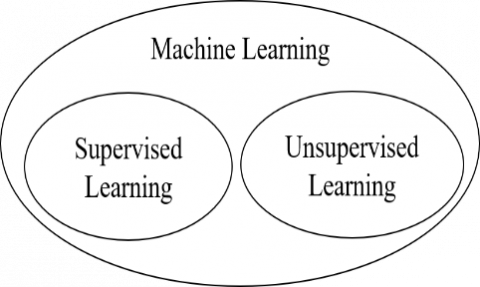Figure 2. Types of machine learning

The remaining sections of this research work are organized as follows. Section-2 discusses ‘Related Work’, Section-3 presents the ‘Methods Used and Proposed Model’, Section-4 describes the ‘Experimental Results and Analysis’. Section-5 discusses ‘Conclusion and Future Scope.

2. Related Work

This section completely discusses the related work done by different researchers in the same area of research.

Yu et al.  have implemented the machine learning techniques by considering the decision tree algorithm of C4.5. The proposed methodology of this work uses 13 features rather than 18 stroke scale features for determining and analyzing the stroke classification. The data collected from the database of the National Institutes of Health Stroke Scale (NIHSS) for the study of cerebrovascular strokes among people affected over age 65 years. Out of total samples, 75% of subjects were used for training, and 25% of subjects were used for testing. The conclusion that in this work, the C4.5 decision tree algorithm has made promising results in determining the criticality and analyzing the classification of the stroke also it had decreased the factors of the stroke from the database of NIHSS features. Based on the hypothetical solutions 91.11% decent accuracy is obtained through the C4.5 decision tree algorithm.

Monteiro et al.  have implemented a machine learning methodology to determine the practical results of the patients affected with ischemic stroke when admitted for three weeks. Among different types of strokes, Ischemic stroke acts as a major purpose of disorder and death all over the world among people of 65 years and in adults. The proposed methodology is succeeded on an outcome of the outlined superior AUC value of 0.808± 0.085 when compared to the foremost point score of 0.771 ± 0.056 with 70% subjects used for training and 30% subjects used for testing. On the other hand, the model keeps on increasing the additional features depending upon particular timing along with the increase in the AUC score by setting the point score of above 0.90. The Baseline feature sets used under experiment -1 produced a ‘good’ outcome with 51.3% and a ‘poor’ outcome with 48.7% accuracies on 425 samples. By obtaining the conclusions and validating the results taken at the time of admission and by making a priority of the use of technological methods whenever required.

Sung et al.  have proposed a methodology that can be examined for automated phenotyping by further classifying the ischemic stroke into 4 subdivisions. This model depending upon the structured and unstructured data taken from the electronic medical records (EMRs). It works on the records of 4640 patients who have been diagnosed with the mild symptoms of Ischemic stroke and also been taken for examining the results. The sub-divisions structured data has National Institutes of Health stroke scale whereas unstructured data has clinical narratives which are refined through a heatmap. The conclusion of stroke scale data from EMRs could make the process clear and smooth phenotyping of ischemic stroke when integrated with the structures data. However, diminishing the different levels of class issues into binary classification work along with the congregation of classifies solution helps in increasing the performance by taking 66% subjects on training and 34% subjects on testing.

Xie et al.  have proposed a model to combine common stroke biomarkers by developing machine learning techniques and to analyze the complete recovery of the ischemic stroke patient within three months. In this work, to predict the recovery terms of the patient Extreme gradient boosting (XGB) and Gradient Boosting Machine (GBM) models were implemented to identify modified ranking scale (mRS) scores by using biomarkers availability within 24 hours of the admitting of the patient. A total of 512 patients records were taken into consideration for analysis with fivefold cross-validation for identifying the improvements of the model. These records are categorized into 80% on training and 20% on testing. Under the binary analysis of an mRS score which is larger than 2 considering biomarkers which are provided during the time of admitting, XGB and GBM include AUC of scores 0.746 and 0.748 accordingly.

Wang et al.  have implemented a machine learning model in the configuration of the risk of symptomatic intracerebral hemorrhage (sICH) after the thrombolysis of the stroke. The risk factors of sICH are theoretically used after stroke thrombolysis. Based on this study, a total of 2578 thrombolysis-treated ischemic stroke patients were recognized from January 2013 and December 2016. Out of which 70% were taken into training modules and 30% considered under nominal data test sets. In order to analyze the risk of sICH, these machine learning modules were helped to increase the performance analysis metrics through the area under curve (AUC) with 0.82.

Lin et al.  have proposed a hybrid neural network model with 10 cross folds for evaluating the stroke outcome. The data collected from “Taiwan Stroke Registry” is given for the model with 70% on training and 30% on testing.

Sung et al.  have implemented machine learning algorithms to analyze the stroke outcome with acute minor stroke. Among 739 patients, 61 patients having a negative outcome after a stroke at 90 days. The data is categorized into 89.4% for no END and the remaining 10.6% for END This database related to patients was taken from NIHSS with a score of ≤ 3. Pre indication of the neurological deterioration tells us that diminishing of the NIHSS score within days of the admission of the patient. The inimical score was determined from the modified Ranking scale score of ≥ 2. In this work, four machine learning models such as bootstrap decision forest, boosted trees, Logistic Regression, and Deep neural network was used in analyzing the early signs of neurological deterioration and examined with a decent accuracy of 94.6%.

In the existing research work, the authors have done only a classification of various types of strokes. This leads to improper classification and we do not attain decent accuracy to predict the stroke severity. Due to this, there is a gap identified for predicting the risk levels of stroke factors which are low, moderate, high and severe. To overcome this limitation, in this research work we have deployed three levels of risk identification hierarchy modules. These modules have been implemented with the help of the proposed algorithm to predict the risk levels of stroke factors along with classification.

3. Methods Used

This section comprises of different benchmark machine learning models with their limitations, the dataset used for this research work, and proposed architecture with its advantage over existing models.

3.1 Machine learning models

3.1.1 Gaussian Naïve Bayes

Supervised learning algorithm uses the Naïve Bayes Model, which depends on Bayes theorem involves resolving the different divisions of the errors. Among different types of Naive Bayes methodologies, we implement “Gaussian naïve Bayes” in this research work. The probabilities of the patients effected by hemorrhagic and ischemic strokes can be written as:

$P\left(\frac{A}{B}\right)=\sum_{w \in A \cap B} P\left(\frac{W}{B}\right)+\sum_{w \in A \cap B^{c}} P\left(\frac{W}{B}\right)$  (1)

$=\sum w \in A \cap B \frac{P(w)}{P(B)}$   (2)

$=\frac{P(A \cap B)}{P(B)}$   (3)

where, ‘A’ is probability of getting stroke and ‘B’ defines highest occurring value of every primary attribute in dataset.

The instances represented by solution vector x = (x1, x2, x3,……,xn) and P(Ck | x1, x2, x3,……,xn ), where, ‘Ck’ defines the ‘kth’ class and ‘k’ is the number of classes.

The conditional probability of Gaussian Naïve Bayes can be decomposed as follows:

$P\left(C_{k} \mid \mathrm{x}\right)=\frac{P\left(C_{k}\right) P\left(\mathrm{x} \mid C_{k}\right)}{P(\mathrm{x})}$   (4)

Using the probability on construction a model classifier is calculated as:

$\hat{y}=\operatorname{argmax}\left\{P\left(c_{1}\right) \prod_{i=1}^{n} P\left(\frac{x_{i}}{C_{1}}\right), P\left(c_{2}\right)\right.$$\left.\prod_{i=1}^{n} P\left(\frac{x_{i}}{C_{2}}\right)\right\}$   (5)

where, ‘n’ defines number of primary parameters (n=9), ‘C1’ defines the low risk and ‘C2’ defines the high risk.

3.1.2 Linear regression

The name itself is referred to as the precise correspondence among the dependent and independent variables considering few or more variables so-called linear regression. Since the linear regression represents in a precise way in its correspondence by predicting the change in the variables of dependent classes to that of the change of the variables in the independent classes is calculated as:

Y = b0 + b1*X1+ b2*X2 + b3*X3 +……. + bn*Xn      (6)

where, ‘Y’ is a dependent variable, X1……Xn are independent variables, b0.….bn are the regression coefficients. The number of attributes ‘n’ is written as:

$Y_{i}=\sum_{i=1}^{n} X n^{*} b_{n}+b_{0}$   (7)

In this research work, we consider the “Multilinear regression” technique from among the various types of linear regression modules. In general, taking the variables than the required sum in the independent class that is required in analyzing the binary dependent values. This process of linear regression is referred to as Multilinear regression and the sum of squares error (SSE) can be calculated as:

$S S E=\sum\left(Y_{i}-Y_{i}^{\mid}\right)^{2}$   (8)

where, Yi is ‘Predicted Output’ and $Y_{i}^{\mid}$ is ‘expected output’.

3.1.3 Logistic regression

Logistic regression comes under the technique of supervised learning, which is used for analyzing the absolute dependent values by making use of the variable among the required blocks of the independent values. Analysis of the output values can be determined by the logistic regression of the absolute dependent values. Hence the solutions can be drawn as the absolute or the differential variables. It may be of any form either numerical or binary variables i.e., Yes or No, 0 or 1, true or false, etc. In the computer-determined language, the values are given in the 0 or a format but this model represents the feasible value that lies between 0 and 1. The usage of the values is the only difference between Logistic regression and Linear Regression. The retrogradation of the problems can be settled using linear regression whereas the categorization of the issues was carried out by the Logistic regressions is written as:

Sigmoid Function $\emptyset(z)=\frac{1}{1+e^{-z}}$   (9)

where, z = b0 + b1 * age + b2 * systolicBP + b3 * diastolicBP + …… + b9 * cholesterol.

3.1.4 K-Means

Combining the group of unlabeled datasets into various forms, that binds the datasets into a collection that comes under an unsupervised learning algorithm that configures in K-Means grouping. In this research work, the variable K is represented as the number of existing groups that are required to be initialized in this technology. This required grouping the datasets into various combined variables from among the various groups and in an easy manner without considering the training process by identifying various datasets in the unlabeled classes in an independent way is calculated as:

$\sum_{j=1}^{k} \sum_{i=1}^{n}\left\|X_{i}^{(j)}-\mathrm{Cj}\right\|^{2}$  (10)

where, ‘k’ is number of clusters, ‘n’ is number of patients and ‘cj’ defines risk factor can be low, medium, moderate, and risk.

3.1.5 Support vector machine

The main aim of this model is to develop the exact linear way or deterministic partition which separates n-proportional space into groups such that providing easy access of combining the data which is newly formed into their respective modules for further references. This type of sorting the data by an exact linear way can also be referred to as hyperplane.

Since considering these outermost vector points that are supportive in building the hyperplane are termed as support points and so the algorithm is named as Support vector algorithm. With the help of the demo graphs that are categorized into two variant ways which are divided considering the deterministic partition or a hyperplane.

The linear SVM is required in linearly differentiating the information/data, that represents dividing the dataset into two different classes by a unique linear separation. Data is termed to as the linear differential and the classifier is written as:

Class 1 (Low risk) $=(\mathrm{W} * \mathrm{X}+\mathrm{b}) \geq 1, \forall \mathrm{X}$   (11)

Class 2 (High risk) $=(\mathrm{W} * \mathrm{X}+\mathrm{b}) \leq-1, \forall \mathrm{X}$   (12)

where, ‘W’ is a vector to Hyper plane, ‘b’ is a bias and ‘x’ is a matrix from dataset.

The non-linear term itself referred to as the in deterministic data division, that represents a dataset that cannot be divided by the shortest route, that particular information is referred to as non-linear data and hence the classifier is written as:

$K(X, Y)=(1+X * Y)^{d}$   (13)

where, ‘X’ is data of low risk, ‘Y’ data of high risk and ‘d’ is degree of the polynomial.

The RBF kernel represents a consequence that gives points relies upon the measured interval from the initial origination or from any particular point is written as:

$K\left(X, X^{1}\right)=\exp \left(-\frac{\left\|X-X^{1}\right\|^{2}}{2 \sigma^{2}}\right)$  (14)

where, || X-X1|| defines the distances between the two risk vectors and let $\gamma=\frac{1}{2 \sigma^{2}}$

$K\left(X, X^{I}\right)=\exp \left(-\gamma\left\|X-X^{1}\right\|^{2}\right)$   (15)

3.1.6 Decision tree

The decision tree consists of two apexes, those are Decision apex and the leaflet apex. Among these, the Decision apex shows the features of the number of limbs attached to it and are required in making decisions, on the other hand, the leaflet apexes represent the outcomes of these decision limbs and they do not have any limbs attached to them. The selection or implementation depends upon the type of dataset that is provided. Depending upon the required scenarios these are represented in the form of a graph by drawing all the possible outcomes based on the selection/complication. Since it starts with the source apex and diverges in various directions by developing a structure of the tree is calculated as:

Gini Index $(\mathrm{G})=\sum_{i=1}^{c} P_{\mathrm{i}}\left(1-P_{\mathrm{i}}\right)$   (16)

where, ‘c’ is number of classes, ‘pi’ defines the probability of class ‘i’, and ‘G’ becomes the root node which having more value.

3.1.7 Random forest

Random forest is based on the theory of ensemble learning. It is a procedure that indulges various classifiers in resolving compounded drawbacks by enhancing the execution of this technology. Considering the average in enhancing in identifying the speed of the particular dataset by the random forest which consisting of multiple decision trees relying on different subset among the given dataset. Alternately rather than depending on a single decision tree, the random forest concludes every single tree and on the maximum identifications by finally displaying the identified outcome. The importance of each feature of a decision tree can be calculated as follows:

$f i_{i}=\frac{\sum_{{ j: node\,j\, splits\, on\,feature\, i }}^{n i_{j}}}{\sum_{k \in {all\, nodes }}^{ n i_{k}}}$                                            (17)

where, ‘fii’ defines the ‘ith’ feature importance, ‘nij’ defines the importance of node ‘j’.

Algorithm 1: Random forest

Step 1: Apply bootstrapping procedure for creating subsets of data.

Step 2: Create decision tree for each subset generated at step 1.

Step 3: Test data given to each decision tree and decision trees produces values.

Step 4: The majority value associated to decision tree can be considered for final prediction.

Boosting is the technique of combining all the weak classifiers under single strong classifiers. During the instruction period, it produces n number of multiple decision trees. Once the final decision tree is formed the data record that has found incorrectly when first identified is considered to be the major priority. Based on this the output of these is gathered and sent for the next further decision model. This process iterates and repeats itself until we attain the required base learners recommended to create at the initial stage.

Step 1: Initialize the weights for all training points by using:

W = $\frac{1}{N}$    (18)

Step 2: Calculate error rate for each “weak classifier” by using:

$\epsilon=\sum_{w e a k} w_{i}$   (19)

Step 3: Select the lowest error rate classifier.

Step 4: Find the $\alpha$ for the classifier by using:

$\alpha=\frac{1}{2} \log \frac{1-\epsilon}{\epsilon}$  (20)

Step 5: Check if the classifier is good or not by using:

$f\left(x_{i}\right)=\sum_{t=1}^{T} \alpha_{t} h_{t}\left(x_{i}\right)$   (21)

Step 6: Update weights of each classifier based on the previous classifiers.

Step 7: Go to step-2 to step-6 for number of iterations ‘T’.

3.1.9 Cross validation

Cross-validation is a technique, where the data is divided into ‘n’ variables depending upon the subsets. Once these variables are attained, the training process is initiated on all the ‘k’ folds except the last variable ‘k-1’ which is sent for the testing process.

3.2 Proposed improved random forest

The improvised random forest model predicts the stroke severity by using three hierarchical modules. The module-1 predicts the risk level of intracerebral and subarachnoid hemorrhagic based on the presence of ischemic stroke. Module-2 predicts the risk level of ischemic and subarachnoid hemorrhagic based on the presence of intracerebral hemorrhagic. Module-3 predicts the risk level of ischemic and intracerebral hemorrhagic based on the presence of subarachnoid hemorrhagic.

Adding to the high accuracy of the improvised random forest model, we have also developed a User Interface (UI) to take in data from the user. The UI ensures better understanding about the details the user needs to give and it also adds a pluggable look and feel to the whole process.

Additionally, our proposed model also has an exponentially better accuracy for predicting the occurrence of brain strokes given the age, Systolic BP, Diastolic BP, BMI, Glucose levels, Cholesterol and any existing smoking habits. We have spiked the accuracy of the system, by using multiple base estimators in the random forest algorithm unlike the single base estimators which are generally used. In addition to the multiple base estimators, we have also used cross folding to explore and find out the best features in the given dataset.

3.3 Proposed model architecture

The proposed model predicts the stroke severity in three different level factors such as low risk, moderate risk, and high risk based on the primary attributes followed by classification technique.

## 3.jpg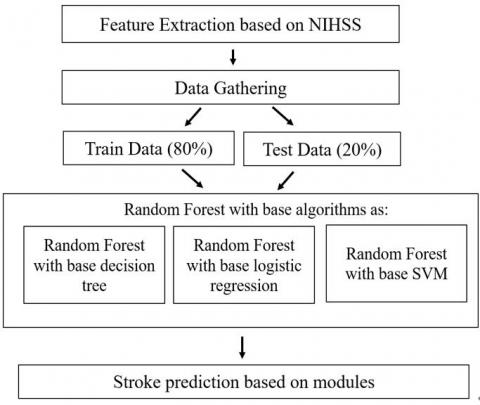Figure 3. Model architecture

Figure 3 shows the proposed model can extract the features based on NIHSS for data gathering processes. After data gathering, the machine learning model works depending on the training data (80%) and testing data (20%). Both the training data and the test data are sent as inputs into the Random Forest algorithm which has the base classifiers namely base decision tree, base logistic regression and base SVM. The risk factor is thereby generated from the above model architecture. The detailed work flow of the proposed architecture is demonstrated in the following steps:

Step 1: Data Collection and pre-processing is explained in Figure 4(a).

## 4a.jpgFigure 4(a). Feature extraction based on stroke scale

Step 2: Risk Formation is explained in Figure 4(b).

## 4b.jpg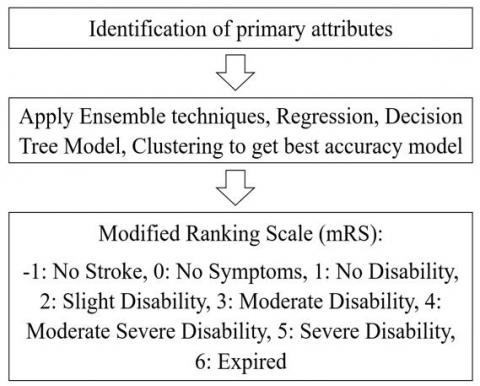Figure 4(b). Risk formation based on best accuracy model

Step 3: Risk identification with different levels of stroke is represented in Figure 4(c).

## 4c.jpgFigure 4(c). Stroke level prediction

3.4 Proposed improvised random forest algorithm

Algorithm 3: Stroke Prediction (SPN)

Step 1: If the model trained is ‘False’ then load the trained data and start training the model

Step 2: From the user data initialize the required data for the prediction.

Step 3: Assign ‘Y’ with a return value of the predicted object.

Step 4: if Y = = 0 then return “Low Risk”

else if Y = = 1 then return “Moderate Risk”

else if Y = = 2 then return “High Risk”

else return “Severe Risk”

Algorithm 4: Model Training

Step 1: Load the trained data.

Step 2: From the user data initialize the trained model object.

Step 3: Return the model object.

Algorithm 5: Stroke predictor (SPR)

Step 1: Load the required new data.

Step 2: Process the new record with the existing model object

Step 3: From the new record, initialize the predicted object.

Step 4: Return the model object with predicted stroke levels.

3.5 Dataset

The data set used in this research work includes a total of 4,799 subjects which contains 3,123 males and 1,676 females and the summary of primary attributes are available in the data set shown in Table 1 . As a part of data pre-processing, from the above available dataset, we have excluded the ‘Gender’ attribute in this research work.

Table 1. Summary of dataset

 Attribute Minimum Maximum Mean Standard Deviation Age NIHSS mRS Systolic BP Diastolic BP Glucose Paralysis Smoking BMI Cholesterol 1 0 -1 100 59 70 0 0 18 160 90 45 6 195 135 295 3 3 45 253 47.12 18.12 3.67 153.09 103.65 225.85 1.36 0.88 33.73 217.53 23.69 11.27 1.87 24.92 18.34 56.11 1.106 0.9 6.23 20.26

3.6 Performance evaluation metrics

In the process of testing the Area Under Curve (AUC) and Receiver Operating Characteristics (ROC) is one of the standardized ways. It deals with the probability among the classifier of randomly picked positive sample to that of the randomly picked negative sample.

Accuracy (ACC) is evaluated to the number of all exact identifications separated to that of the sum of a number of the dataset.

Accuracy = $\frac{\text { True Positive }+\text { True } \text { Negative }}{\text { True Positive }+\text { True } \text { Negative }+\text { False Negative }+\text { False Positive }}$

The Sensitivity is evaluated to the number of identifications of correct positive divisions to that of the total positives number.

Sensitivity =$\frac{\text { True Positives }}{\text { True Positives + False Negative }}$

The Specificity is determined by the total amount of correct negative identifications separated by the total negative numbers.

Specificity =$\frac{\text { True Negative }}{\text { True Negative + False Positives }}$

The False Positive Rate is determined by the FP / (FP+TN) evaluation. It is determined to the ratio of negative points that are wrongly taken as positive when compared to that of all the negative points of the data.

False Positive Rate =$\frac{\text { False Positive }}{\text { True } \text { Negative }+\text { False Positive }}$

F1-Score determines how specific the classifier is and also how rigid it can be. The proportion of high precision and low recall draws out the most accurate possibilities, but it omits various examples that become complex while dividing. The mathematical expression is given by:

F1- Score = 2 $* \frac{1}{\frac{1}{\text { precision }}+\frac{1}{\text { recall }}}$

The Precision gives multiple positive outcomes when distinguished by a number of positive results identified by the classifiers.

Precision $=\frac{\text { True Positives }}{\text { True Positives + False Positives }}$

4. Results and Discussion

This section shows the performance results among benchmark machine learning models and stroke level prediction model (improved random forest) depending up on the metrics as follows:

4.1 Performance evaluation of machine learning models

From Table 2, out of these machine learning algorithms, the Random Forest classifier was found out to be the best performer with an accuracy of 94.23%, 92.16% sensitivity, 95.07 specificities, 0.04% low error rate results. So, we chose the Random Forest classifier to make our prediction algorithm and improvised it to increase the prediction accuracy. The performance analysis of benchmark models is shown in Figure 5.

Table 2. Performance results of Machine Learning Models

 Model Accuracy Sensitivity Specificity Decision Tree Gaussian Naïve Bayes Logistic Regression Linear SVM Poly SVM RBG SVM Random Forest AdaBoost AdaBoost with SGD 93.12 76.77 82.5 83.22 83.43 81.97 94.23 86.87 78.02 91.84 83.25 93.23 95.33 94.20 96.13 92.16 95.78 96.86 93.75 64.89 62.83 61.06 63.71 56.04 95.07 72.47 40.68

## 5.jpgFigure 5. Performance analysis of machine learning models

4.2 Comparison results of basic and improvised random forest

In the basic random forest, we have solely relied on one single base estimator. That might give an erroneous and a less accurate result. In order to enhance the accuracy, we have proposed an improvised random forest algorithm which uses three base estimators to improvise and have a much better refined result. To prove the same, we have showcased the results of both the basic and proposed random forests in Table 3.

## 6.jpg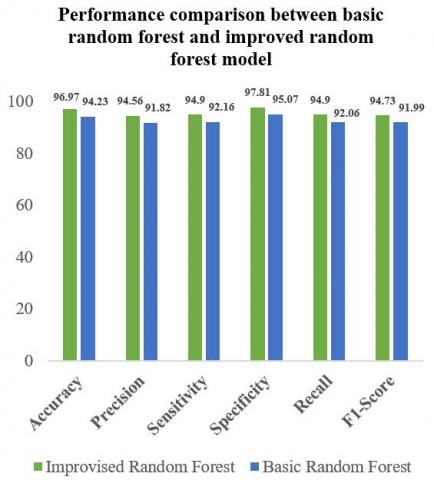Figure 6. Performance analysis between existing and proposed model using random forest

By using the proposed model, we can identify the risk level of stroke along with the classification of disease levels with an accuracy of 96.97%. Table 3 demonstrates the performance between existing Random Forest and improvised Random Forest models. The visual representation of performance metrics is shown in Figure 6.

Table 3. Performance metrics of proposed model with risk levels using improvised random forest

 Performance Metrics Basic Random Forest Improvised Random Forest Accuracy Precision Sensitivity Specificity F1-Score Error rate 94.23 91.82 92.16 95.07 91.99 0.04 96.97 94.56 94.9 97.81 94.73 0.03

This best accuracy model is given for SPN algorithm to find out the risk levels of stroke such as low, moderate, and high based on the three-level of modules.

Also, the SPN algorithm using the proposed model generates important features based on the primary attributes scores available in the dataset that can be ischemic, intracerebral, and subarachnoid hemorrhagic are shown in Figure 7.

## 7.png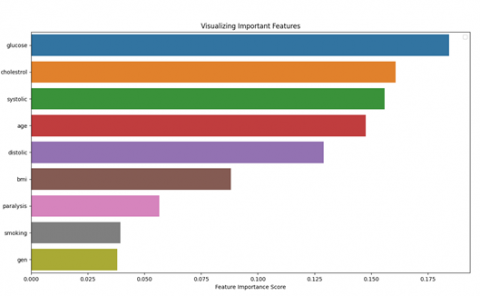Figure 7. Feature importance score using proposed model

## 8.jpgFigure 8. SPR model UI for predicting risk factors

The stroke level prediction model initialized with primary attributes given by the user to find the risk levels. In Figure 8, the user can enter a new record that will be processed with the existing data. In the new record, a patient already diagnosed with ischemic stroke showing symptoms of abnormal blood pressure levels. Due to this abnormality and earlier stroke, module-1 of the proposed model can predict the chance of occurring the risk level of remaining strokes that can be intracerebral or subarachnoid hemorrhagic. The Receiver Operating Characteristics (ROC) curve for the stroke prediction model between basic and improvised random forest is shown in Figure 9.

## 9.jpg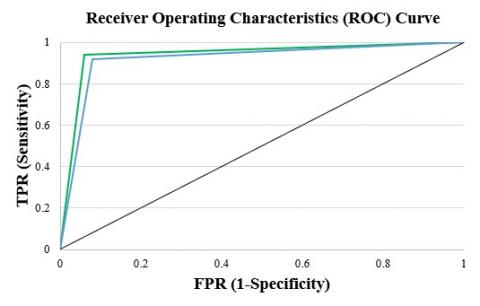Figure 9. ROC for stroke predictor model

5. Conclusion and Future Scope

An improvised Random Forest ensemble technique with a stroke prediction algorithm is implemented in this research work to identify the risk factor. An accuracy of 96.97% is achieved through the stroke predictor (SPR) model with an error rate of 0.03%. Using an improvised Random Forest model, we obtained efficient results with improved prediction accuracy. As future research, we can derive methods for different types of strokes along with risk levels using an image data set.

Acknowledgement

I sincerely thank Dr. Debnath Bhattacharyya for guiding us to support this research work and also thank Dr. Akif Ahmad, M.B.B.S & Dr. D. Jagan Mohan Rao, M.B.B.S for their valuable guidance.

References

 http://www.strokecenter.org/patients/about-stroke/stroke-statistics/united-states, accessed on 24 October, 2020.

 https://www.ncbi.nlm.nih.gov/pmc/articles/PMC4782523, accessed on 24 October 2020.

 https://www.stroke.org.uk, accessed on 25 November 2020.

 Wang, S., Li, Y., Tian, J., Peng, X., Yi, L., Du, C., Feng, C., Liang, X. (2020). A randomized controlled trial of brain and heart health manager-led mHealth secondary stroke prevention. Cardiovascular Diagnosis and Therapy, 10(5): 1192-1199. https://doi.org/10.21037/cdt-20-423

 Harrar, D.B., Salussolia, C.L., Kapur, K., Kleinman, M.E., Mannix, R., Rivkin, M.J. (2019). A stroke alert protocol decreases the time to diagnosis of brain attack symptoms in a pediatric emergency department. The Journal of Pediatrics, 216: 136-141.E6. https://doi.org/10.1016/j.jpeds.2019.09.027

 Sun, F., Liu, H., Fu, H.X., Li, C.B., Geng, X.J., Zhang, X.X., Zhu, J., Ma, Z., Gao, Y.J., Dou, Z.J. (2020). Predictive factors of hemorrhage after thrombolysis in patients with acute ischemic stroke. Frontiers in Neurology, 11: 1309. https://doi.org/10.3389/fneur.2020.551157

 Wilkinson, D.A., Daou, B.J., Nadel, J.L., Chaudhary, N., Gemmete, J.J., Thompson, B.G., Pandey, A.S. (2020). Abdominal aortic aneurysm is associated with subarachnoid hemorrhage. Journal of Neuro Interventional Surgery. https://doi.org/10.1136/neurintsurg-2020-016757

 Rasmussen, M., Valentin, J.B., Simonsen, C.Z. (2020). Blood pressure thresholds during endovascular therapy in ischemic stroke-reply. JAMA Neurology, 77(5). https://doi.org/10.1001/jamaneurol.2020.3819

 Lattanzi, S., Silvestrini, M. (2016). Blood pressure in acute intra-cerebral hemorrhage. Annals of Translational Medicine, 4(16): 1-2. https://doi.org/10.21037/atm.2016.08.04

 Verma, A., Jaiswal, S., Sheikh, W.R. (2020). Acute thrombotic occlusion of subclavian artery presenting as a stroke mimic. Journal of the American College of Emergency Physicians Open, 1(5): 932-934. https://doi.org/10.1002/emp2.12085

 Boukobza, M., Nahmani, S., Decschamps, L., Laissy, J.P. (2019). Brain abscess complicating ischemic embolic stroke in a patient with cardiac papillary fibroelastoma - Case report and literature review. Journal of Clinical Neuroscience, 66: 277-279. https://doi.org/10.1016/j.jocn.2019.03.041

 Uppal, S., Goel, S., Randhawa, B., Maheshwary, A. (2020). Autoimmune-associated vasculitis presenting as ischemic stroke with hemorrhagic transformation: A Case report and literature review. Cureus, 12(9): e10403. https://doi.org/10.7759/cureus.10403

 Convertino, V.A., Moulton, S.L. (2011). Use of advanced machine-learning techniques for noninvasive monitoring of hemorrhage. The Journal of Trauma, 71(1): S25-S32. https://doi.org/10.1097/TA.0b013e3182211601

 https://www.potentiaco.com/what-is-machine-learning-definition-types-applications-and-examples, accessed on 19 September 2020.

 Yu, J., Park, S., Lee, H., Pyo, C.S., Lee, Y.S. (2020). An elderly health monitoring system using machine learning and in-depth analysis techniques on the NIH stroke scale. Mathematics, 8(7): 1-16. https://doi.org/10.3390/math8071115

 Monteiro, M., Fonseca, A.C., Freitas, A.T., Melo, T.P., Francisco, A.P., Ferro, J.M., Oliveira, A.L. (2018). Using machine learning to improve the prediction of functional outcome in ischemic stroke patients. IEEE/ACM Transactions on Computational Biology and Bioinformatics, 15(6): 1953-1959. https://doi.org/10.1109/TCBB.2018.2811471

 Sung, S.F., Lin, C.Y., Hu, Y.H. (2020). EMR-based phenotyping of ischemic stroke using supervised machine learning and text mining techniques. IEEE Journal of Biomedical and Health Informatics, 24(10): 2922-2931. https://doi.org/10.1109/JBHI.2020.2976931

 Xie, Y., Jiang, B., Gong, E., Li, Y., Zhu, G., Michel, P., Wintermark, M., Zaharchuk, Z. (2019). Use of gradient boosting machine learning to predict patient outcome in acute ischemic stroke on the basis of imaging, demographic, and clinical information. American Journal of Roentgenology, 212(1): 44-51. https://doi.org/10.2214/AJR.18.20260

 Wang, F., Huang, Y., Xia, Y., Zhang, W., Fang, K., Zhou, X., Yu, X., Cheng, X., Li, G., Wang, X., Luo, G., Wu, D., Liu, X., Campbell, B.C.V., Dong, Q., Zhao, Y. (2020). Personalized risk prediction of symptomatic intracerebral hemorrhage after stroke thrombolysis using a machine-learning model. Therapeutic Advances in Neurological Disorder, 13: 1-10. https://doi.org/10.1177/1756286420902358

 Lin, C.H., Hsu, K.C., Johnson, K.R., Fann, Y.C., Tsai, C.H., Sun, Y., Lien, L.M., Chang, W.L., Lin, C.L., Hsu, C.Y., Registry, T.S. (2020). Evaluation of machine learning methods to stroke outcome prediction using a nationwide disease registry. Computer Methods and Programs in Biomedicine, 190: 105381. https://doi.org/10.1016/j.cmpb.2020.105381

 Sung, S.M., Kang, Y.J., Cho, H.J., Kim, N.R., Lee, S.M., Choi, B.K., Cho, G. (2020). Prediction of early neurological deterioration in acute minor ischemic stroke by machine learning algorithms. Clinical Neurology and Neurosurgery, 195: 105892. https://doi.org/10.1016/j.clineuro.2020.105892

 Bandi, V., Midhunchakkaravarthy, D., Bhattacharyya, D. (2020). Stroke_Analysis. Mendeley Data. https://doi.org/10.17632/jpb5tds9f6.1